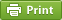Werner's Blog — Opinion, Analysis, Commentary
Interaction effects in regression analysis

Empirical research often involves estimating equations that have interaction effects. Consdier the following simple linear regression $y_i = \alpha + \beta x_i + \gamma z_i +\epsilon_i$ where the dependent variable $$y_i$$ depends on two regressors $$x_i$$ and $$z_i$$, and where the error term $$\epsilon_i$$ is assumed i.i.d. Assume that we can estimate the three parameters with ordinary least squares. After careful review of the underlying theoretical structure, the researcher decides to investigate the interaction effect and estimate $y_i = \alpha + \beta x_i + \gamma z_i + \delta x_i z_i +\epsilon_i$ The problem that arises is that we cannot easily compare the magnitudes of $$\beta$$ and $$\gamma$$ across the two specifications because of the interaction effects. Simply "eyeballing" the numbers will leave us none the wiser because $\frac{\partial y}{\partial x}=\beta+\delta z \quad\mathrm{and}\quad \frac{\partial y}{\partial z}=\gamma+\delta x$ If we compare results, we probably want to compare $$\beta$$ from the first specification with $$\beta+\delta\bar{z}$$ in the second specification, where $$\bar{z}$$ is the average of the $$z_i$$'s in the data set.

In the specification with the interaction effect, we effectively estimate an intercept and a slope. Depending on the range and scale of the $$z_i$$, the intercept can be quite large. So what can a researcher do to make estimation results comparable across the different specifications that involve interaction effects? In case of a linear specification, the answer is quite simple: de-mean the key variables and estimate $y_i = \alpha_1 + \beta_1 (x_i-\bar{x}) + \gamma_1 (z_i-\bar{z}) +\epsilon_i$ and $y_i = \alpha_2 + \beta_2 (x_i-\bar{x}) + \gamma_2 (z_i-\bar{z})+ \delta_2(x_i-\bar{x})(z_i-\bar{z}) +\epsilon_i$ Now compare $$\partial y/\partial x=\beta_1$$ from the first regression equation with $$\partial y/\partial x=\gamma_2+\delta_2(z-\bar{z})$$ from the second regression equation. As we want to compare again at midpoint $$z=\bar{z}$$, we find $$\partial y/\partial x=\gamma_2$$ evaluated at $$z=\bar{z}$$. Now we can simply compare $$\beta_1$$ with $$\beta_2$$ and see how much it is different across the two specifications. We can do the same with $$\gamma_1$$ and $$\gamma_2$$. De-meaning can be a useful trick to maintain a level of coherence across different estimation specifications. This makes most sense when there is a need to compare the results across the specifications. Technically, the estimates are all isomorphic, but the interpretation is made much easier if the estimates are all around the midpoints of the regressors.

If you use Stata, demeaning is particular easy. You can simply create a new variable that is demeaned:

// Demeaning variable x egen mean_x = mean(x) generate x_m = x - mean_x

If you have to demean multiple variables, you can also use a Stata program and then pass the names of the variables to the program. Just make sure all the variables are numeric. The program replaces the original variable with the demeaned variable.

// Demean multiple variables using a simple program program define demean foreach var of local 0 { sum var', meanonly replace var'=`var'-r(mean) } end // demean variables x and z demean x z
Posted on Tuesday, March 1, 2016 at 09:45 — #EconometricsRecent Blog Entries

Topics

Months

Subscribe to RSS feed© 2023  Prof. Werner Antweiler, University of British Columbia.